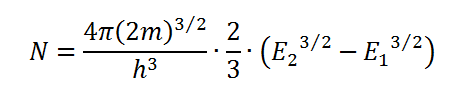# Density of states per unit volume in a solid Calculator

This is a CalcTown calculator to calculate the density of states per unit volume in a given energy range in a solid

meV
meV

#### Result

states/m-3where

m is the mass of electrons

h is the Planck's constant